# Hydrodynamic Voltammetry

## 1. Introduction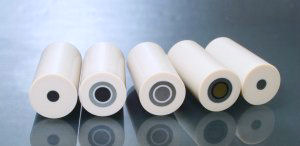As the electrode reaction for the chemical species in solution occurs on the interface of solution (liquid phase) and electrode (solid phase), in general it proceeds through three elementary steps:
1) substrate mass transfer to the electrode surface;
2) electron transfer reaction on the electrode surface;
3) product diffusion from the electrode.
Electrode reaction rate (current) is considered to be controlled by the slowest process. It is very important to understand the electrode performance controlling process. For example, if the activity of the fuel cell catalyst itself is high enough (the electron transfer reaction is fast), the cell performance can be greatly improved by designing a highly efficient mass transfer electrode (GDE).
Utilizing the rotating disk electrode (RDE) system can control the mass transfer rate for 1) and 3) processes, so that the electrode activity 2) can be quantitatively analyzed in sufficient reproducibility 1-2).
By combining a ring electrode with a disk electrode, the rotating ring disk electrode (RRDE) measurement can help to detect RDE reaction products, their intermediates etc., so that the detailed reaction mechanism can be analyzed3).
In this technical note, while the detailed theoretical formula and analyzing method was omitted, the experiment for RDE & RRDE, such as apparatus using for RDE & RRDE measurement and the basic experimental methods were mainly described. Moreover, the method acquiring the limiting diffusion current data, and Levich and Kouteck-Levich plots preparation method were introduced in brief.

## 2. RRDE-3A Rotating Ring Disk Electrode Apparatus

The feature of hydrodynamic voltammetry (RDE, RRDE) is carrying out electrochemical measurement while working electrode is rotating. In order to obtain the mass transfer rate in good reproducibility, it is very important to keep the electrode rotating in a stable speed during the measurement. Hereby, we would like to introduce our new product electrode rotator RRDE-3A Rotating Ring Disk Electrode Apparatus. If RRDE-3A Rotating Ring Disk Electrode Apparatus is equipped with a rotating disk electrode (RDE) (shown in the Figure 1 upper left), the disk working electrode can rotate during electrochemical measurement.
A rotating ring disk electrode (RRDE) is shown in lower left of Figure 1. The disk electrode is in the center position, and a thin insulating layer and a ring electrode locate around the disk electrode in the shape of a concentric circle. When the RRDE electrode is set up to the RRDE-3A Rotating Ring Disk Electrode Apparatus, the rotating ring disk electrode measurement can be carried out easily. If you have the RRDE-3A Rotating Ring Disk Electrode Apparatus, you can do both RDE and RRDE measurements easily.

 The simple specifications for RRDE-3A Rotating Ring Disk Electrode Apparatus are described as follows: 1. Rotation range: 100 ∼ 8000 rpm 2. Rotation accuracy: < 0.1% 3. Power supply: 100 - 240 VAC; 50/60 Hz 4. Operating temperature: 10 - 50°C 5. Inlet gas pressure: 5 psi maximum 6. Size (W x D x H): 190 × 230 × 380 mm 7. Weight: 6 kg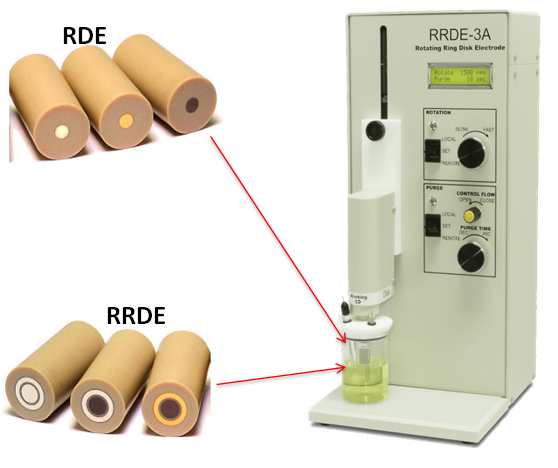Figure 1: RRDE-3A is used for both RDE (Rotating Disk Electrode) and RRDE (Rotating Ring Disk Electrode) for measurements.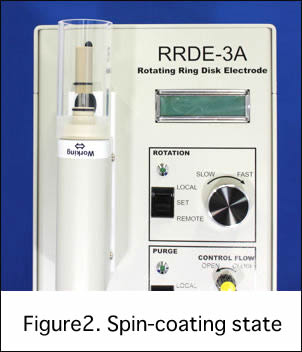Besides the normal rotation function, the motor portion can be upside down so that the spin coating can be easily done if an acrylic pipe is used together. This function is quite convenience for the electrode modification with catalyst.
Furthermore, gas purge function is equipped in RRDE-3A Rotating Ring Disk Electrode Apparatus, the manual purge time control is available certainly, it works with ALS electrochemical analyzer, and the remote purge time control is also possible.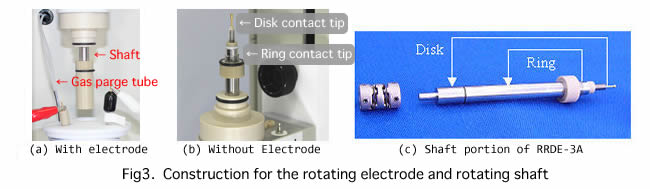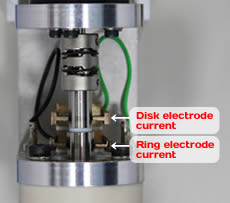Electric contact between rotating electrode and potentiostat is performed through the silver carbon brushes contacting to the rotation shaft. Figure 3(a) shows the shaft attached with electrode. The electrode can be removed easily by twisting with fingers. You can see the shaft without electrode in Figure 3(b), and the disk and ring tip parts are insulated by PEEK. The disk tip contacts with the disk electrode, so the disk current goes through the shaft center and flows to the top of the shaft, as shown in Figure 3(c). The ring tip contacts with the ring electrode and the ring current flows to the middle of the shaft. By using two pairs of the carbon brush contacting to the upper and middle parts of the shaft (Figure 4), the ring and disk currents can flow to the potentiostat during electrodes rotating. If the carbon brushes are worn, the noise will occur during measurement. If you find serious noise, please check the carbon brush firstly. If wearing of the carbon brushes is too serious, please replace them.

## References

1) A.J. Bard and L. R. Faulkner. Electrochemical Methods Fundamentals and Applications 2nd Edition, Wiley, New York (2001).
2) K. Oyaizu, M. Yuasa, et al. Electrochemistry, 73, 1060 (2005).
3) K. Oyaizu, M. Yuasa, et al. Electrochemistry., 74, 81 (2006).

## 3.Rotating disk electrode (RDE) measurement

### 3.1. Feature and measurement of RDE instrument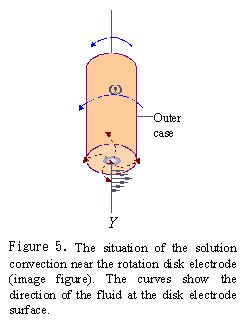[Feature of RDE instrument]
When RDE electrode is rotated in the solution, the spinning disk drags the fluid at its surface and, because of centrifugal force, flings the solution outwards from the center in a radial direction. The fluid at the disk surface is replenished by a flow normal to the surface. The convection occurs near the disk surface.
Because there is a thin layer rotating together with the disk electrode, the convection velocity of solution from the disk surface to distance Y is distributed as shown in Fig.5. The convection velocity of the solution is improved with the increasing of the rotation rate, as a result, the diffusion layer becomes thin and diffusion rate is increased. It means that the diffusion rate of substrate is regulated by controlling the electrode rotation rate.
For normal static electrode measurement, such as cyclic voltammetry, in order to analyze mass transfer process and electron transfer process, the potential scan rate is changed. The peak current difference can be found under different scan rates.
One feature of the RDE is the smallest concentration variation of the substrate near the electrode surface, so the current does not depend on time and shows a constant value. In the case of RDE measurement, under some potential scan rate (dependent on systems studied, usually, tens of mV/s), the diffusion limiting current is independent of scan rate. Hysteresis between positive scan and negative scan almost can not be found. The current-potential curve obtained using RDE is called hydrodynamic voltammogram.

[Apparatus used for RDE Measurement]
The instruments required for RDE measurement are listed below:
a) Potentiostat (e.g. ALS600C or 700C electrochemical analyzer)
b) Personal computer for potentiostat control (generally Windows PC)
c) counter electrode (Pt winding wire)
d) reference electrode (suitable type for the electrolyte)
e) rotating disk working electrode (e.g. ALS RDE, disk electrode portion of RRDE electrode)
f) cell for RDE
g) electrode rotator (e.g. ALS RRDE-3A)
a)∼d) are the same as what are used in the usual electrochemistry measurement, such as cyclic voltammetry. e)∼g) are special equipments for RDE measurement.

### 3.2. Experiment for fast electron transfer redox pair [Fe(CN)63−/Fe(CN)64−]

The redox reaction with electrode surface electron transfer rate higher enough than the substance diffusion rate is called a reversible system.

Fe(CN)63− + e ⇔ Fe(CN)64-            Eq. 1

[Fe(CN)63−/Fe(CN)64−] is a typical reversible system. In generally, a reversible system for RDE measurement can help to obtain the diffusion coefficient D of substrate, reversible half peak potential E1/2 and electron transfer number n.

[Electrolyte Preparation]
2 mM K3[Fe(CN)6] including 0.1 M KNO3 was prepared for the measurement. High purity reagents were dissolved in pure water (nano-purity or distilled water). The concentration of substrate K3FeCN6 should be prepared exactly. Please pre-electrolyze the supporting electrolyte solution beforehand using the method reported in literature4 if the measurement time is long.

[Setup for disk electrode]
a) Electrode surface was polished with alumina and rinsed with pure water before use.
b) The electrode was set to the shaft.
c) Turned shaft with hand and checked whether the electrode surface was rotated horizontally without eccentricity.
d) The rotation number was changed from 500 rpm to 5000 rpm to confirm whether there was any shaking or abnormal noise.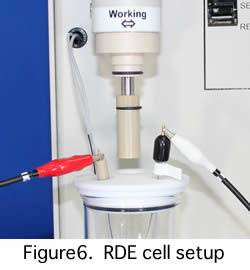[RDE cell]
a) Glass cell and Pt counter electrode were washed with water and rinsed with pure water. After rinsing with a small amount of electrolyte solution, the electrolyte solution was poured to cell up to predetermined level.
b) The reference electrode (Fig.6) beforehand rinsed with pure water was set up.
c) Working electrode was dipped into the solution and adjusted to the proper height and center position of cell cover, so that the turbulent flow might not occur.
d) Nitrogen gas was used for purge (about 20 ∼ 30 minutes) to remove dissolved oxygen (If you use RRDE-3A, manual and remote purge time control are available). Please remember to adjust the nitrogen gas to minimum flow rate before measurement.

[Connection and setup for electrode rotator and potentiostat]
All leads of electrode rotator and potentiostat including the leads to reference electrode (Ag/AgCl) and counter electrode were connected. After finishing above preparation, make sure no air bubbles in disk electrode surface and reference electrode liquid-liquid junction part.

[CV measurement with RDE electrode]
After setting the instrument, the power source turns on and starts the ALS software window of PC. CV measurements with scan rate changing in 100, 50, 25,10 mV/s and CV plots are recorded simultaneously.

[CV judgment]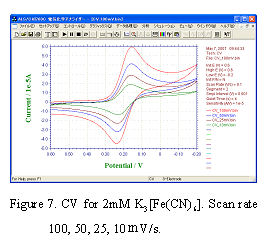CV curves are shown in Fig.7. The feature for reversible system describes in below:
a) Potential for peak current is independent of scan rate.
b) The difference of anode peak potential and cathode peak potential is 59 mV/n (n is the number of reaction electron) at 25°C.
c) Peak current is proportional to v1/2.
CV curves of Fig.7 fulfill above conditions mostly, and the RDE electrode surface is considered to be normal. If the electrode is found to be dirty , electrochemical cleaning is required. If the dirty can not be still removed, please exchange the solution and examine the same operation. If the problem is still there, don’t be hurry. It is better to re-polish the electrode and re-prepare the solution.

[Measurement for Hydrodynamic Voltammetry]
a) Scan rate was set to 10 mV/s and segment was set to 1, the parameters were same to CV.
b) The electrode rotated at e.g. 500 rpm and rotation stability was checked.
c) The potential scans to negative direction(0.6 V ∼ -0.2 V) and data record in the same procedure as CV measurement. The rotation rates change (e.g. 500, 1000, 2000, 3000, 4000, 5000 rpm) and corresponding current-potential voltammograms record.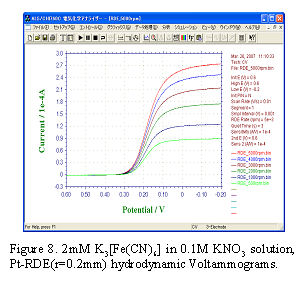[Data analysis – Levich plot]
Fig.8 shows hydrodynamic voltammograms. If cathodic polarization is enough (in this case E < 0 V), diffusion limited current (or limiting current) iL can be observed.
iL is proportional to square root of electrode angular rotation rate (ω1/2) as shown in Eq.2.

iL = 0.62 n F A D 2/3 ω1/2 ν-1/6 C            Eq.2

F is faraday constant (C/mol), A is the electrode surface area (cm2/s), ω is the electrode angular rotation rate (rad/s), v is the kinetic viscosity (cm2/s) of solution, C is the bulk concentration of substrate (mol/cm3). The relationship for angular rotation rate ω to rotation rate f (rpm) is ω = 2πf/60.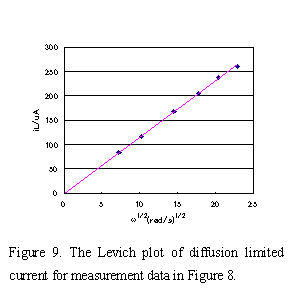iL currents obtained under various rotating rate were plotted versus ω1/2 and a straight line (Levich plot) which pass though the zero point was obtained in Fig.9. The diffusion coefficient D, the value of reaction electron n, etc. can be calculated from the slope. At 25°C pure water value of 0.01 cm2/s was used as kinetic viscosity of solution, and number of reaction electron n = 1, C = 2 x 10-6 mol/cm3, A = 0.1256 cm2 were used for equation (5). The diffusion coefficient D = 6.73 x 10-6 (cm2/s) of [Fe(CN)6]3- could be obtained from the slope. If the electrochemical reaction is accompanied by preceding or the succeeding chemical reaction, the plot will deviate from the straight line.

### 3.3. Experiment for slow electron transfer system (oxygen reduction)

The slow electron transfer process on the electrode surface is called irreversible system (It does not mean the reverse reaction not occurring). An example of the typical reaction is the reduction of dissolved oxygen in acidic solution.

O2 + 2e + 2H+ → H2O        Eq.3
O2 + 4e + 4H+ → 2H2O        Eq.4

The information obtained from RDE measurement for irreversible system are kinetically-controlled current and the catalyst electron transfer number Napp, which can be calculated from the slope of a Koutecky-Levich plot in the case of electrode catalyst.

i-1 = ik-1 + (0.62 n F A D2/3ω1/2v-1/6C)-1        Eq.5

Eq.5 is called Koutecky-Levich equation. The first term on the right side of equation is activity determining current which is not dependent on rotation rate. The second term is a reciprocal of Eq.2 and means the mass transfer resistance depending on rotation rate.

[RDE measurement for reduction of oxygen]
Pt working electrode (disk part of Pt-Pt RRDE electrode polishes with alumina and cleaned before use) sets to RRDE-3A, and Ag/AgCl reference electrode and Pt winding wire counter electrode put into the cell. The electrolytes used were 0.2 M Na2SO4 and 0.1 M H2SO4, the saturated oxygen solution was obtained by purging the oxygen gas for 30 minutes. At first, the potential was scanned on the electrode in stationary state and the proper potential scan range (0.8 V ∼ -0.2 V) was determined. Next, the RDE measurement was carried out at scan rate 25 mV/s while the electrode was rotating and the voltammogram was recorded simultaneously (Fig.10).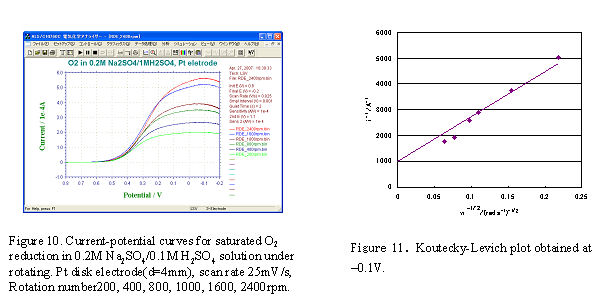The currents corresponding to a fixed potential (-0.1 V) for various rotation rates in Fig.10 were plotted vs. ω-1/2, a straight line was obtained in Fig.11. Using the dissolved oxygen concentration (C = 1.27 × 106 mol/cm2), O2 diffusion coefficient (Do = 2.0 × 10-5 cm2/s), solution kinetic viscosity (v =0.01 cm2/s), electrode surface area (A = 0.125 cm2), and faraday constant (F = 96,485 C/mol), the reaction electron number n = 3.6 (4 electrons reaction) can be obtained from the slope value.

## References

4) M. Watanabe, Denki kagaku (presently Electrochemistry),53, 671 (1985).
5) C.Paliteiro, A. Hamnett and J.B. Goodenough, J. Electroanal. Chem., 233, 147 (1987).

## 4. Rotating ring disk electrode (RRDE) measurement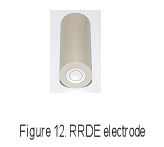### 4.1. Feature for rotating ring disk electrode (RRDE)

RRDE electrode, composed of ring and disk electrodes, is used for RRDE-3A, as shown in Fig.12. The electrode rotation is controlled by RRDE-3A, and both potentials (ED and ER), from ring and disk electrode, are independently controlled by a common reference electrode and counter electrode. The currents are recorded by a dual potentiostat (700C series electrochemical analyzer).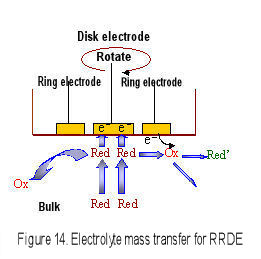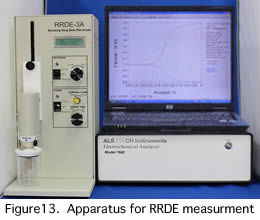When the RRDE electrode is rotated, the convection occurs near the electrode surface, therefore the diffusion layer thickness is constant and the diffusion limited current is observed. This feature is the same to RDE (Levich equation is used for reversible system). The advantage for RRDE is that the electrolytic product in disk electrode is transported to the ring electrode by centrifugal force and is detected in ring electrode. The electrochemical reaction mechanism in disk electrode is analyzed in more detail.

### 4.2. RRDE collection efficiency calculation and measurement using reversible system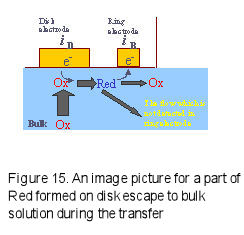In order to perform quantitative RRDE measurement, it is necessary to understand the electrolytic species transport situation from disk electrode to ring electrode. The typical parameter is Collection Efficiency N.
If the below reactions occur in disk and ring electrodes, the Red species formed ion the disk electrode are oxidized at the ring electrode, which potential has been set to the Red oxidation potential and can be detected as a ring current.
However, a part of Red species generated on the disk electrode may escape to the bulk during transport from the disk electrode to the ring electrode (Fig.15), and the |iD| > |iR| relation can be found. The collection efficiency is defined as a ratio of the absolute value of ring current to disk current (Eq. 6).

Disk electrode: Ox + e- → Red (reduction)
Ring electrode: Red - e- → Ox (Oxidation)
Collection efficiency N = |iR|/|iD|                                                                                Eq.6

The collection efficiency is a constant determined only by relative configuration and size of both the electrodes and can be calculated using the theoretical equations shown below.

N = 1 - F(α/β) + β2/3[1 - F(α)] - (1 + α + β)2/3 {1 - F[(α/β)(1 + α + β)]}                       Eq.7
α = (r2/r1)3 - 1                                                                                                           Eq.8
β = (r3/r1)3 - (r2/r1)3                                                                                                    Eq.9
F(θ) = [31/2/(4π)]ln[(1 + θ1/3)3/(1 + θ)] + [3/(2π)] arctan[(2θ1/3 - 1)/31/2] + 1/4           Eq.10

r1 is the radius of a disk electrode, r2 and r3 are the inside and outside radius of a ring electrode, respectively. If r1 = 0.2 cm, r2 = 0.25 cm and r3 = 0.35 cm are used, the calculated collection efficiency is 0.424.
Because an exact electrode surface is uneven, the real collection efficiency is hardly to be coincidence to the theoretical calculation value. In generally, reversible systems such as [Fe(CN)6]4−/[Fe(CN)6]3−, hydroquinone/benzoquinone, ferrocene0/+ , Br/Br3and so on are used for RRDE electrode collection efficiency measurement.
Let's see an example for collection efficiency measurement. A Pt ring-Pt disk electrode (r1 = 0.2 cm, r2 = 0.25 cm, r3 = 0.35 cm) was used for RRDE measurement. The RRDE electrode was dipped into 2 mmol/L K3[Fe(CN)6] in 0.1 mol/L KNO3solution and rotated under a rotation rate (e.g. f = 300, 500,……, 5000 rpm). Disk potential ED was scanned from 0.6 V to -0.2 V vs. Ag/AgCl at scan rate 10 mV/s, ring potential ER was fixed to 0.6 V (the reduced product [Fe(CN)6]4- can be oxidized at this potential), and the current-potential voltammograms were recorded during the electrode rotation. Fig.16 shows the RRDE measurement. The ratio of |iR-L| / |iD-L| is almost constant under various ω. The average of collection efficiency N is 0.422, this value is quite near to the calculated N value (0.424).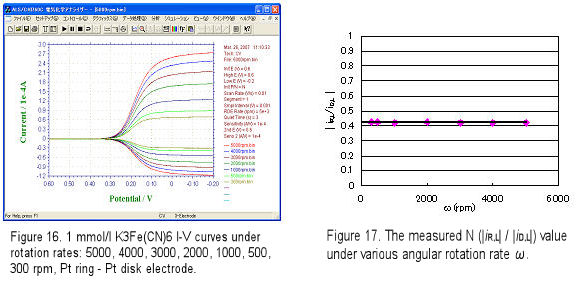### 4.3 Oxygen reduction measurement using Pt ring-GC disk RRDE electrode

Pt ring (ID = 5 mm, OD = 7 mm)- GC disk (D = 4 mm) RRDE (polished by alumina and cleaned before use) electrode was set to the RRDE-3A rotator and put into the cell which was equipped with Ag/AgCl reference and Pt counter electrodes. Electrolyte was 1 M NaOH and the saturated oxygen solution was obtained by purging O2 gas for 30 minutes (purge time was controlled by RRDE-3A remote mode). The potential was scanned under static state to understand the proper potential range for the GC disk electrode (potential range changes with the electrode material).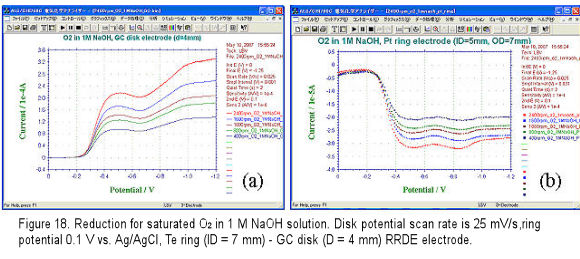For RRDE measurement, the disk potential scan rate was set to 25 mV/s (depend on experiment, 10, 5 mV/s are also used), the ring potential was set to 0.1 V so that the oxygen reduction product H2O2 formed at disk electrode can be oxidized and detected. The voltammograms were recorded while potential negative scan and electrode rotation. The obtained disk and ring current voltammograms are shown in Fig.18(a) and (b), respectively.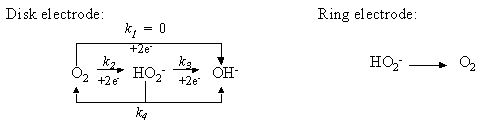In generally, the oxygen reduction occurred on carbon material in alkaline solution is expressed in above equations. When potential is scanned to negative, the oxygen receives 2 electron (k2) and is changed to HO2-. If the potential is scanned to more negative, furthermore 2 electron reaction (totally 4 electrons) occurs (k3) and OH- is formed, the current increases and the second wave appears. Moreover, the chemical reaction for HO2- disproportionation into O2 and OH- also occurs (k4). Ring potential is set to 0.1 V so that the formed OH- is oxidized completely and detected as ring current.
At -0.5 V disk potential, the disk current of Fig.18(a) increased and the corresponding ring current in Fig.18 (b)also increased. However, when the disk potential was scanned to more negative e.g. -1.0 V, the ring current decreased. This indicated that some of HO2- ion formed on the disk electrode was further reduced to be OH- and caused the ring current decreasing. This means by detection in ring electrode, disk reaction product and reaction mechanism are analyzed. The data in Fig.18 are not so ideal; in reference5) when a Pt ring- pyrolytic graphite (edge plane) disk electrode was used, the second wave 4 electrons reduction proceeded completely, and k1k2 and k3 were analyzed quantitatively.
In recent years, cathodic catalytic reduction of oxygen relating to fuel cell research has attracted much attention. Platinum or a platinum alloy is often used as electrode catalyst for fuel electrode or oxygen electrode. Research works regarding platinum replacement catalyst are active in order to lower fuel cell development cost6). For example, 4-or 2-electrons oxygen reduction occurred on disk electrode in an alkaline solution. The 2-electrons reduction intermediate HO2- was transported to the ring electrode by rotation and was detected at the ring electrode by oxidation (Fig.19).
Fig. 19 Image for the catalytic reaction occurs on the rotating ring disk electrode.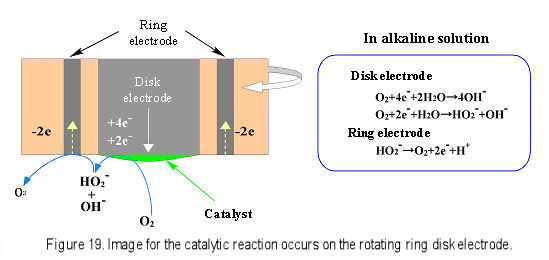In reference 6), the oxygen reduction using Ag-W2C catalyst modified on the disk electrode was proved to be 4-electrons reaction by analyzing the ring current. Furthermore, reciprocal current i-1 was plotted to ω-1/2, and Koutecky-Levich plot was obtained. The calculation for slope confirmed that the Ag-W2C was 4-electrons reduction catalyst same to the platinum. Through above RRDE investigation, the platinum substitution catalyst Ag-W2C was estimated to be an outstanding oxygen reduction catalyst.

## 5. Conclusion

Hydrodynamic voltammetry RDE and RRDE measurements can obtain the constant current by electrode rotation causing convection near the disk surface and controlling the diffusion layer thickness. Electrochemical reaction mechanism for reversible and irreversible systems can be analyzed by changing the rotation rate. Especially, RRDE can be used for studies of wide range applications7-10) such as fuel cell catalyst reaction, metal, alloy dissolution and plating mechanism analysis, electroorganic synthesis, optical reaction, etc. As the hydrodynamic voltammetry RDE and RRDE can help to acquire much electrochemical information, the much more applications in the future are expected.

### References

5) C.Paliteiro, A. Hamnett and J.B. Goodenough, J. Electroanal. Chem., 233, 147 (1987).
6) H. Meng, P.K. Shen, Electrochemistry Communication, 8, 588, (2006).
7) T. Ousaka, S. Oyama, T. Ohsaka, Electrochemical Methods, Koudansha, Japan (1989).
8) A. Fujishima and K. Honda, Denki Kagaku (Electrochemistry), 42, 213 (1974).
9) M. Watanabe and H. Uchida, Electrochemistry, 68, 816 (2000).
10) K. Tokuda and T. Ohsaka, Denki Kagaku (Electrochemistry), 61, 193 (1993).

##### We dispatch worldwide

For purchases that exceeds \$100

##### OFF

Discount Electrodes